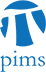## Number Theory Seminar: Computing conjectural integers to extreme precision

• Date: 03/04/2010
Lecturer(s):
Tom Boothby (University of Washington)
Location:

University of British Columbia

Description:

According to the Birch and Swinnerton-Dyer conjecture, the order of the Tate-Shafarevich group can be expressed as the ratio of several invariants of the curve. However, this ratio involves two numbers which are expected to be irrational; namely $L^{(r)}(E,1)$ and $\Omega_E$. In the case $r >= 2$, this ratio has never been proven to be rational. Recently, we have provably computed the ratio (1.000...) to 10kbits of precision for such a curve. We present a provable algorithm to compute $L''(E,1)$ with $O(p^3)$ runtime, where $p$ is the desired precision.

Schedule:

5:10 - 6:00pm, WMAX 110.